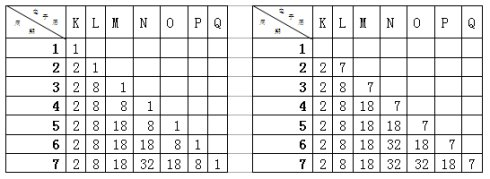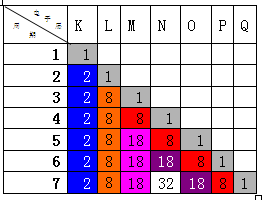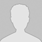#学习小专题

## 同一主族的两种元素的原子序数之差到底有多少组解

（2005年全国理综卷第9题）：同一主族的两种元素的原子序数之差不可能是（ ） A 16 B 26 C 36 D 46 （2006广东，2）同主族两种元素原子核外电子数差值可能为（ ） A. 6 B. 12 C. 26 D. 30 笔者在组织学生复习处理以上两题时，很多学生都是采用的验证法，认为这种题型思

（2005年全国理综卷第9题）：同一主族的两种元素的原子序数之差不可能是（

A  16       26            36          46

（2006广东，2）同主族两种元素原子核外电子数差值可能为（

A.         B.  12        C.  26        D.  30因每1周期未填充元素，所以应先去掉最外层相同的电子数和相同的K层电子数，再依此类推，还可以补充四组数值，分别为：8+18+32+32+18=108；18+32+32+18=100；18+32+32=82；32+32=64。

 周期 1 2 3 4 5 6 7 容纳元素的种类数 2 8 8 18 18 32 32

由此可知，同主族元素的原子序数差值为：2、8、18、32；

2+8=10、2+8+8=18（重复）、2+8+8+18=36、2+8+8+18+18=54、2+8+8+18+18+32=86、2+8+8+18+18+32+32=118（目前元素种类数不足118可以忽略）；

8+8=16、8+8+18=34、8+8+18+18=52、8+8+18+18+32=84、8+8+18+18+32+32=116（因目前元素种类数不足118可以忽略）；

8+18=26、8+18+18=44、8+18+18+32=76、8+18+18+32+32=108；

18+18=36（重复）、18+18+32=68、18+18+32+32=100；

18+32=50、18+32+32=82；

32+32=64。

同理可知共有21组解。分别为：2、8、10、16、18、26、32、34、36、44、50、52、54、64、68、76、82、84、86、100、108。

(责任编辑：化学自习室)

------分隔线----------------------------

------分隔线----------------------------

 稀有气体定位法：由原子序数推断 元素原子序数的确定方法文档 原子序数与化学式关系归纳 元素原子序数的确定方法 元素倒置之谜 确定元素原子序数的方法几例 为什么氟氯溴碘随着原子序数的增 用左手快速推算元素位置和原子序• 全部评论（0
还没有评论，快来抢沙发吧！